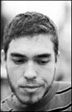## DSP tutorial: RTTY decoder using FFT & DPLL

This example is still a basic RTTY decoder, similar to the previously discussed one, the main difference is that it looks for the start bit and keeps bit sync using a simple but efficient DPLL algorithm. Like before, it uses the oversample & FFT & average method for bit decision making.

#### Problems with decoding using FFT

Note that this method would work well if we could increase the oversample count to more higher rates (decrease the size of the chunks).

The main problem is that if you use even such a low bitrate as 45.45 bauds, and 48kHz sampling rate, one bit is only 1056 samples long. If you are oversampling 4 times, one chunk will be only 1056/4 = 264 samples long. It means that you will have 264 bins after the FFT, representing the 0-24kHz range, so one bin will hold the spectral power of a 24000/264 = 91 Hz wide band.

If you are using the standard 170 Hz shift for RTTY decoding this means you have the two carriers in neighboring bins. So if you increase the oversampling, or decrease the sample rate the two carriers will be in the same bin and you won’t be able to separate them.

#### The DPLL algorithm

The algorithm is described in detail in the RTTY decoding using an IIR filter section of my tutorials. In this case it uses chunks instead of samples (the demodulator returns bit values of chunks).

## 1 Comment »Jacek2019-12-11 12:08:50

Can your code be used to decode RTTY baud = 200
data bits = 7
stop bits = 2. I tried to modify your code but without success. Any advice?

Name (required)
E-mail (required - never shown publicly)
Webpage URL
Comment:
You may use <a href="" title=""> <abbr title=""> <acronym title=""> <b> <blockquote cite=""> <cite> <code> <del datetime=""> <em> <i> <q cite=""> <s> <strike> <strong> in your comment.